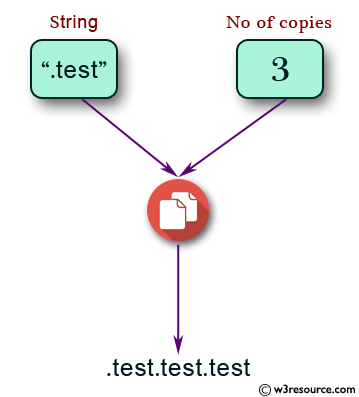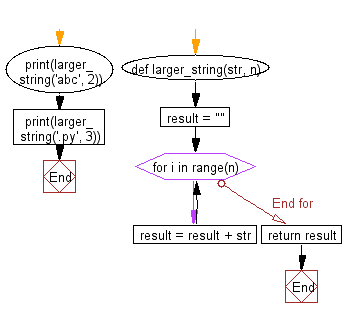﻿ Python: Get a string which is n (non-negative integer) copies of a given string - w3resource# Python: Get a string which is n (non-negative integer) copies of a given string

## Python Basic: Exercise-20 with Solution

Write a Python program to get a string which is n (non-negative integer) copies of a given string.

Pictorial Presentation:Sample Solution:-

Python Code:

``````def larger_string(str, n):
result = ""
for i in range(n):
result = result + str
return result

print(larger_string('abc', 2))
print(larger_string('.py', 3))
```
```

Sample Output:

```abcabc
.py.py.py
```

Flowchart:## Visualize Python code execution:

The following tool visualize what the computer is doing step-by-step as it executes the said program:

Python Code Editor:

Have another way to solve this solution? Contribute your code (and comments) through Disqus.

What is the difficulty level of this exercise?

Test your Python skills with w3resource's quiz

﻿

## Python: Tips of the Day

Unpack variables from iterable:

```# One can unpack all iterables (tuples, list etc)
>>> a, b, c = 1, 2, 3
>>> a, b, c
(1, 2, 3)

>>> a, b, c = [1, 2, 3]
>>> a, b, c
(1, 2, 3)
```